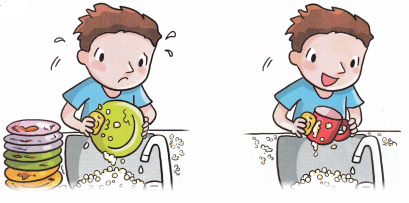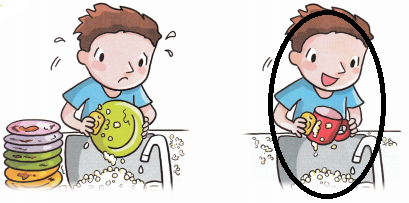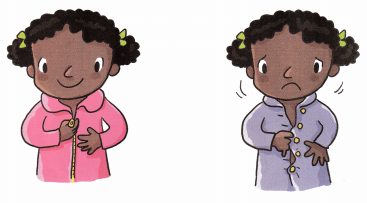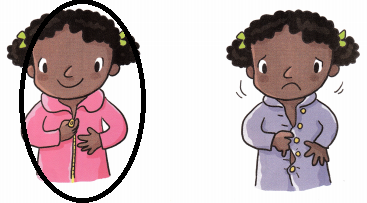# Math in Focus Kindergarten Chapter 19 Answer Key Measurement

Go through the Math in Focus Grade K Workbook Answer Key Chapter 19 Measurement to finish your assignments.

## Math in Focus Kindergarten Chapter 19 Answer Key Measurement

Lesson 1 Comparing Weights Using Nonstandard Units

Circle the heavier thing.

Question 1.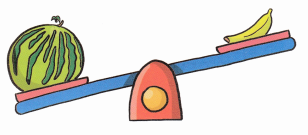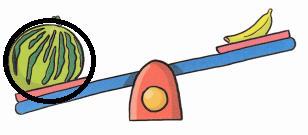Explanation:
The watermelon is heavier than banana

Question 2.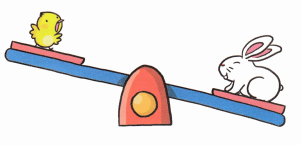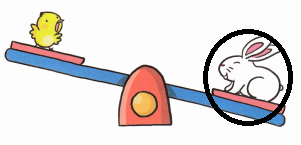Explanation:
The rabbit is heavier than bird

Question 3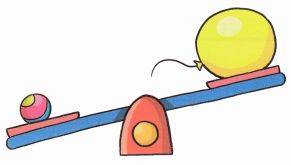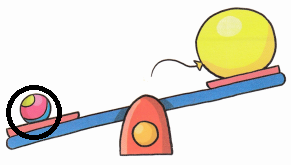Explanation:
The ball is heavier than a balloon

Circle the lighter thing.

Question 1.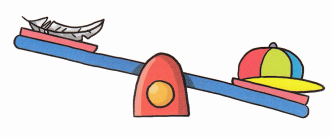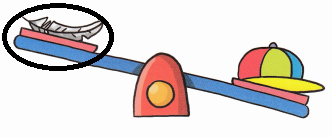Explanation:
The Feather is lighter than the cap

Question 2.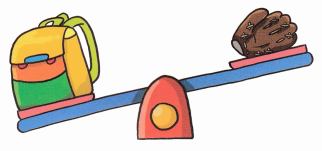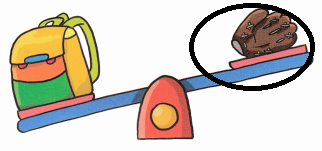Explanation:
The Gloze is lighter than the bag

Question 3.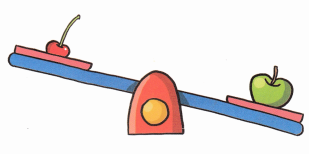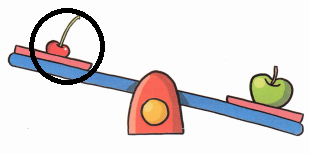Explanation:
The berry is lighter than the apple

Count and write.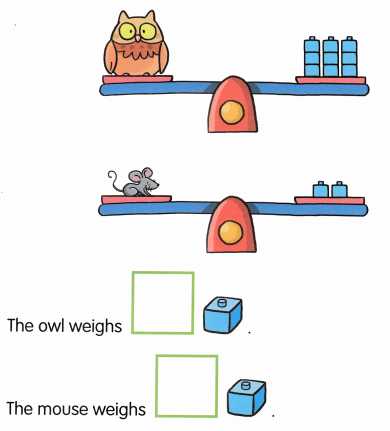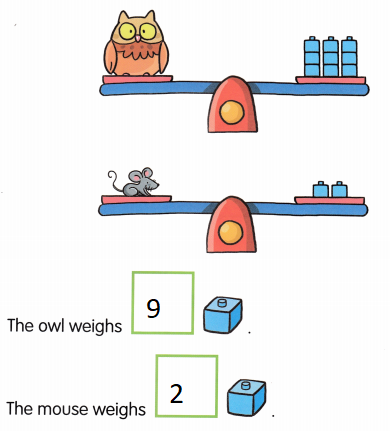Explanation:
The owl weighs 9 blocks and the mouse weighs 2 blocks

Circle the heavier animal.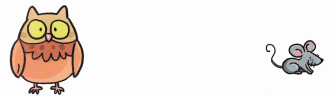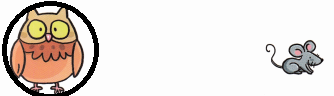Explanation:
The owl weighs more than the mouse

Count and write.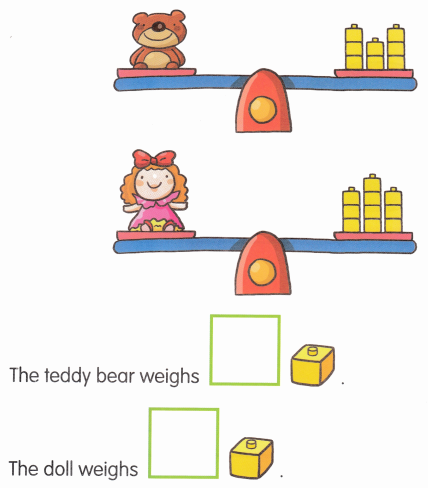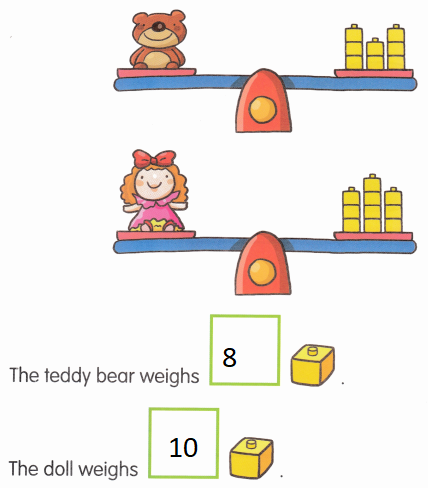Explanation:
The teddy bear weighs 8 blocks and  doll weighs 10 blocks

Circle the lighter thing.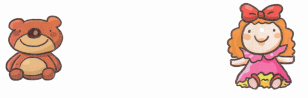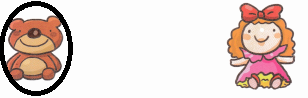Explanation:
The teddy weighs lighter than the doll.

Lesson 2 Comparing Capacities

Circle the container that holds more.

Question 1.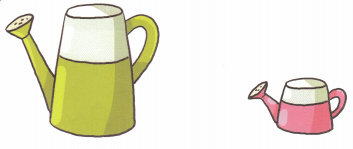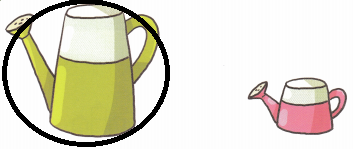Explanation:
The yellow container can contains more than the pink

Question 2.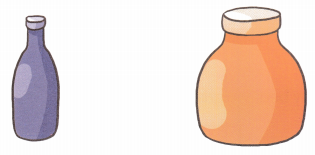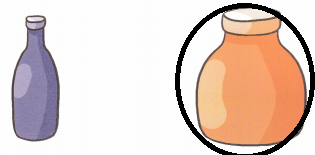Explanation:
The Brown container contains more than the purple.

Question 3.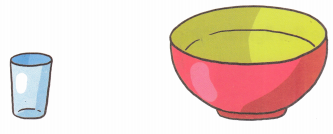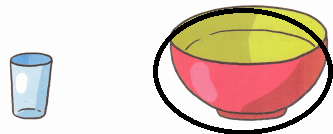Explanation:
The bowl contains more than the glass

Circle the container that holds less.

Question 1.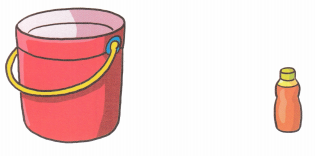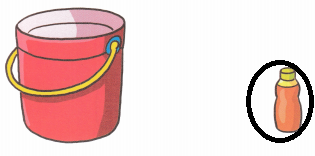Explanation:
The bottle contains less than the bucket

Question 2.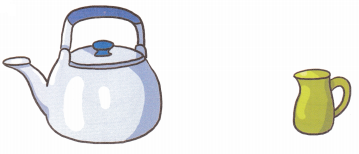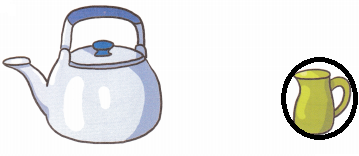Explanation:
The jar contains less than the kettle

Question 3.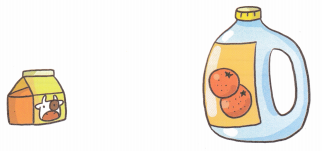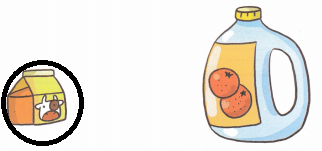Explanation:
The pack contains less than the can

Color the containers that hold the same amount.

Question 1.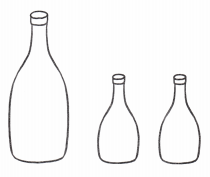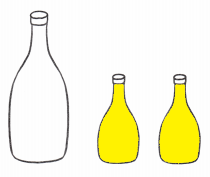Explanation:
The 2 yellow jars contains the same quantity

Question 2.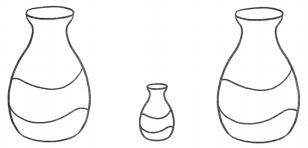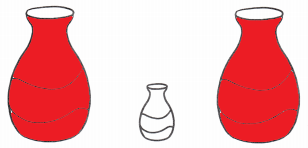Explanation:
The 2 red jars contains the same quantity

Question 3.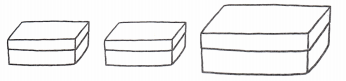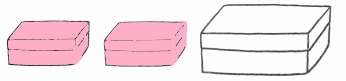Explanation:
The 2 pink boxes contains the same quantity

Lesson 3 Comparing Events in Time

Which takes more time? Circle.

Question 1.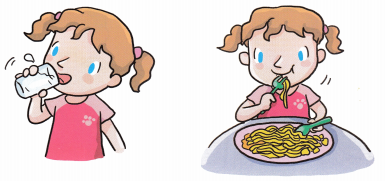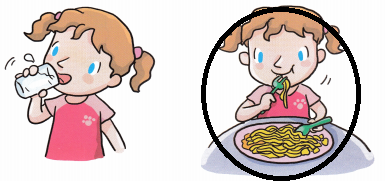Explanation:
Eating takes more time than drinking

Question 2.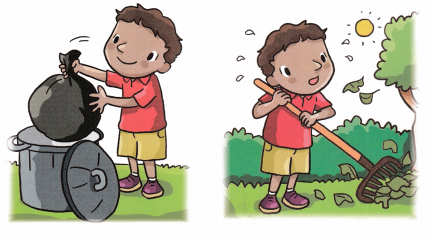Explanation:
Cleaning takes more time than throwing the dust in dust bin

Which takes less time? Circle.

Question 1.